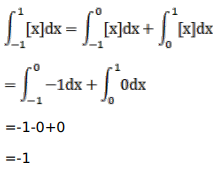# Mark against the correct answer in the following:Question:

Mark $(\sqrt{ })$ against the correct answer in the following:

Let $[x]$ denote the greatest integer less than or equal to $x$.

Then, $\int_{-1}^{1}[x] d x=?$

A. $-1$

B. 0

C. $\frac{1}{2}$

D. 2

Solution: